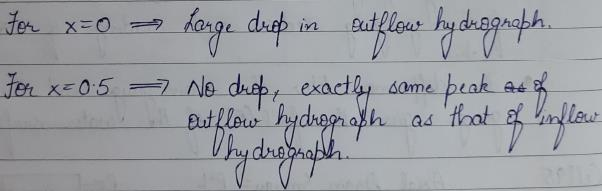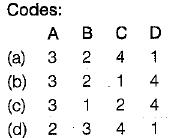Courses

# Flood Routing - 2

## 9 Questions MCQ Test Mock Test Series for Civil Engineering (CE) GATE 2020 | Flood Routing - 2

Description
This mock test of Flood Routing - 2 for Civil Engineering (CE) helps you for every Civil Engineering (CE) entrance exam. This contains 9 Multiple Choice Questions for Civil Engineering (CE) Flood Routing - 2 (mcq) to study with solutions a complete question bank. The solved questions answers in this Flood Routing - 2 quiz give you a good mix of easy questions and tough questions. Civil Engineering (CE) students definitely take this Flood Routing - 2 exercise for a better result in the exam. You can find other Flood Routing - 2 extra questions, long questions & short questions for Civil Engineering (CE) on EduRev as well by searching above.
QUESTION: 1

Solution:
QUESTION: 2

### In case of channel routing, the storage is a function of

Solution:

In reservoir routing, storage is a function of outflow discharge only.

QUESTION: 3

### In the Muskingum method of channel routing if x = 0.5, it represents an outflow hydrograph

Solution:QUESTION: 4

Match List-I with List-ll and select the correct answer using the codes given below the lists:
List-I
A. Reservoir routing
B. Hydraulic routing
C. Channel routing
D. Hydrologic routing

List-ll
1. Solution of equation of continuity
2. Solution of St. Venant’s equations
3. Storage is a unique function of outflow discharge
4. Storage is a function of inflow as well as outflowSolution:
QUESTION: 5

If the storage S, inflow rate I and outflow rate Q for a river reach is written as
S = K[x In + (1 - x) Qn]

Solution:
QUESTION: 6

If I = inflow rate, Q = outflow rate, and S = storage, in a reach the continuity equation used in flood routing is

Solution:
QUESTION: 7

A linear reservoir is one in which

Solution:
QUESTION: 8

An isochrone is a line on the basin map

Solution:
QUESTION: 9

Which of the following equation is used in hydrological flood routing?

Solution: